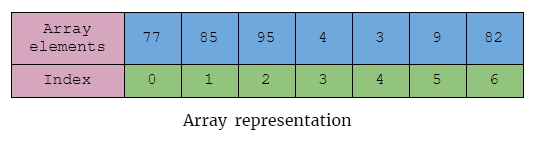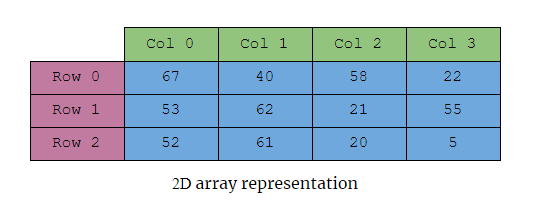×

Search anything:

# 2D arrays in C++ (2 ways)

#### Software Engineering C++In this article, we have discussed what are 2 Dimensional (2D) arrays and what are the different ways we can initialize them and how we can use them in C++.

Table of content:

1. Introduction to array
2. Implementing array in C++
3. 2D arrays using native array
4. 2D arrays using array containers
5. Accessing array elements in 2D array
6. Difference between Native arrays and Array containers

# Introduction to array

Arrays are considered to be one of the basic and useful data structures in any language not just in C++. Hence, before we jump into 2 Dimensional arrays let's just quickly recall some important points about arrays.

An array data structure is a collection of elements stored in contiguous memory locations in a compute under a single variable name.

• Each element in an array can be accessed by its position in the array called as index or key that usually starts from 0.
• All the elements in an array are of the same data type.We know that arrays can be of any data type like integer, float, double, strings, etc. But what if we store arrays inside an array?

Your right! that's when we get a 2-dimensional array.

In short, a 1 Dimensional array is like a list and 2 Dimensional array is like a Table.

# Implementing array in C++

We know that arrays can be implemented in two ways in C++

1. Native arrays - like the arrays in the C language
``````int arr;
``````

Native array

1. Using the array container in C++
``````std::array<int , 10> arr;
``````

Array container

Note: To use the array container we must include the array header file in c++.

Now let's see how we can implement 2D arrays using both these methods.

# 2D arrays using native array

The syntax of native 2 dimensional arrays is similar to one dimenisonal arrays.

``````data_type array_name[n][m];
``````

Syntax of 2D native array

Here,

• data_type - refers to what type of data is going to be stored in this 2D array
• array_name - the name of the array given by the user
• n - Number of rows
• m - Number of Columns

2D arrays can be initialized in different ways lets see them with an example.

``````int arr;
``````

Consider an example - a 2D array with 3 rows and 4 columns

It can be initialized in the following ways,

``````// initializes an 2D array of size 3*4 with garbage values
int arr;
``````

This puts random garbage values inside the 2D array

``````// initializes an 2D array of size 3*4 with 0 as default value for all
int arr = {};
int arr {};
``````

This method of initialization assigns 0 for all the values in the 2D array.

``````// initializes an 2D array with 12 values given from the user
int arr = { {67, 40, 58, 22}, // row 0
{53, 62, 21, 55}, // row 1
{53, 62, 21, 55}  // row 2
};

int arr = {67, 40, 58, 22, 53, 62, 21, 55, 53, 78, 15, 61};
``````

Here, In the second method, the nested parenthesis is neglected but both the lines of code does the same job of initializing a 2D array with user desired values.

``````// initializes an 2D array with 4 values form the user and 0 for the remaing values
int arr = { 67, 40, 58, 22 };
``````

This method creates a 2D array with some of the values from the user and the remaining values are set as 0.

``````// intializing without 1st subscript (i.e the number of rows)
int arr[] = {67, 40, 58, 22, 53, 62, 21, 55, 53, 78, 15, 61};
``````

In the method the 1st subscript i.e. the number of rows is not mentioned but the compiler decides by itself the number of rows needed. Thus creating a 2D array of size 3*4.

# 2D arrays using array containers

Initialization of array containers are just the same as the native arrays, but with a slight change in the syntax

Syntax of the 2D array container

``````array < array<data_type, m>, n > array_name ;
``````

Syntax

Here,

• data_type - is the type of data the 2D array container is going to hold
• array_name - is the name of the array given by the user
• n - number of rows
• m - number of columns

In this syntax, `array<data_type, m>` represents each row in the 2D array, and as whole it represents the whole 2D array itself. This will be clear when we see the example.

``````#include <iostream>
#include <array>
using namespace std;

int main(){

array<array<int, 4>, 3> arr;

return 0;
}
``````

As said before we need to include array header file to use these array containers

Here, in the above code, we are initializing a 2D array container that has 3 array containers in it ( i.e. 3 rows ) and each row has 4 integer values in it (4 columns), hence we get a 2D array container that can hold 12 integer values in total.

The 2D array containers can also be initialized in different ways similar to the native arrays

``````// initializes an 2D array container with garbage values
array<array<int, 4>, 3> arr;

// initializes an 2D array container of size 3*4 with 0 as default value for all
array<array<int, 4>, 3> arr = {};
array<array<int, 4>, 3> arr {};

// initializes an 2D array container with 12 values given from the user
array<array<int, 4>, 3> arr = {67, 40, 58, 22, 53, 62, 21, 55, 53, 78, 15, 61};

array<array<int, 4>, 3> arr = { { {67, 40, 58, 22}, // row 0
{53, 62, 21, 55}, // row 1
{53, 62, 21, 55}  // row 2
} };
``````

Here, in the last method, the values are enclosed by an additional set of parenthesis.

Note: Unlike native array initialization we cannot skip the size of the array container.

``````array< int , > arr ;  // invalid
array< array<int, 4 >, 3>  arr ; // invalid
``````

The above declarations are invalid.

# Accessing array elements in 2D array

Accessing or getting the values from a 2D array is the same for both native arrays and array containers, but array containers have an additional member function to access the elements.

The array elements can be accessed using the `[]` operator along with the array name. The syntax for accessing elements from the 2D array is given below

``````syntax : array_name[row_no][col_no]
``````

Note: Don't forget that indexing starts from `0` rather than `1`.

Let's look into an example,

``````// native 2D array
int arr = { {67, 40, 58, 22}, // row 0
{53, 62, 21, 55}, // row 1
{52, 61, 20, 5}  // row 2
};

cout << arr ; // prints the element 21
``````

To get a better understanding of how the elements are accessed, take a look at the image given below.Similarly, the elements in the array containers can be accessed.

``````// 2D array container
array<array<int, 4>, 3> arr = {{ {77, 85, 95, 4}, // row 0
{3, 9, 82, 91},   // row 1
{6, 43, 25, 95}  // row 2
}};

cout << arr ; // prints the elment 25
``````

But, the array container has a member function called `at()` to access the elements

``````// 2D array container
array<array<int, 4>, 3> arr = {{  {77, 85, 95, 4}, // row 0
{3, 9, 82, 91},  // row 1
{6, 43, 25, 95}  // row 2
}};

cout << arr.at(2).at(2); // prints the elment 25
``````

Both the `[]` operator and `at()` does the same job of getting the element we need from an array but the specialty of `at()` is that it checks if the index is a valid index and prints error if its invalid, while the `[]` operator prints out some garbage value if it is not a proper index of the array.

# Difference between Native arrays and Array containers

When we consider the native array vs array containers there isn't a big difference between the two except in the ways they are used. The `std::array` container has additional member functions which can make our lives a lot easier when it comes to arrays. These additional member functions give a little edge for array containers over the native arrays.

Apart from the functions, the array containers provide have value semantics but the native arrays i.e. you can copy array containers, you can also receive them by value, reference them as function arguments and you can return them by value.

If you are not going to copy the array containers, then there is no other performace difference between the array containers and native arrays.

With this article at OpenGenus, you must have a strong idea of 2D array in C++.